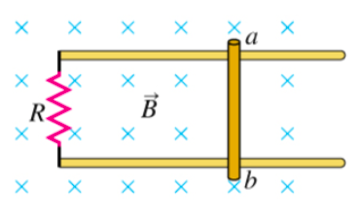# Problem: A 1.30 m long metal bar is pulled to the right at a steady 5.7 m/s perpendicular to a uniform, 0.730-T magnetic field. The bar rides on parallel metal rails connected through R = 25.6Ω, as shown in the figure, so the apparatus makes a complete circuit. You can ignore the resistance of the bar and the rails.Part A. Calculate the magnitude of the emf induced in the circuit.Express your answer using two significant figures.Part B. Find the direction of the current induced in the circuit.a. clockwiseb. counterclockwisePart C. Calculate the current through the resistorExpress your answer using two significant figures.

###### FREE Expert Solution

Part A.

Motional emf:

$\overline{){\mathbf{\epsilon }}{\mathbf{=}}{\mathbf{B}}{\mathbf{l}}{\mathbf{v}}}$ where B is the magnetic field, l and v is the length of the bar and its speed respectively.

B = 0.730T

86% (153 ratings)###### Problem DetailsA 1.30 m long metal bar is pulled to the right at a steady 5.7 m/s perpendicular to a uniform, 0.730-T magnetic field. The bar rides on parallel metal rails connected through R = 25.6Ω, as shown in the figure, so the apparatus makes a complete circuit. You can ignore the resistance of the bar and the rails.

Part A. Calculate the magnitude of the emf induced in the circuit.